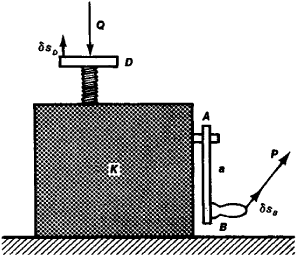# Virtual Work Principle

## Virtual work principle

The principle stating that the total virtual work done by all the forces acting on a system in static equilibrium is zero for a set of infinitesimal virtual displacements from equilibrium. The infinitesimal displacements are called virtual because they need not be obtained by a displacement that actually occurs in the system. The virtual work is the work done by the virtual displacements, which can be arbitrary, provided they are consistent with the constraints of the system. See Constraint

The principle of virtual work is equivalent to the conditions for static equilibrium of a rigid body expressed in terms of the total forces and torques. That is, the principle of virtual work can be derived from these conditions, and conversely. See Equilibrium of forces, Statics

One advantage of the principle of virtual work is that it can serve as a basis for all of statics. In the solution of problems the principle of virtual work is often useful for eliminating the need for consideration of the forces of constraint, since these forces often are perpendicular to the virtual displacements and consequently do no work.

McGraw-Hill Concise Encyclopedia of Physics. © 2002 by The McGraw-Hill Companies, Inc.
The following article is from The Great Soviet Encyclopedia (1979). It might be outdated or ideologically biased.

## Virtual Work Principle

one of the variational principles of mechanics determining the general condition of equilibrium of a mechanical system. According to the virtual work principle, for the equilibrium of a mechanical system with ideal constraints it is necessary and sufficient that the sum of works δAi due to all active forces applied to the system in any virtual displacement be equal to zero. Mathematically, the virtual work principle is expressed by the equation

(1) Σδ·Ai≡ΣFiδsi cos α=0

where the Fi are the operating active forces, the δsi are the magnitudes of virtual displacements at the points of application of these forces, and the αi are the angles between the directions of forces and the virtual displacements. For systems with several degrees of freedom, equation (1) must be formulated separately for each independent dis-placement.

Thus, the virtual work principle makes it possible to find the conditions of equilibrium of a system without introducing unknown constraint forces, which significantly simplifies the solution and expands the class of problems that can be solved. For example, it is easy to find the conditions of equilibrium of a lifting device whose parts are hidden in box K of Figure 1 using this principle. From equation (1) we obtain

Pδsb—Qδ sD=0

where P and Q are the acting active forces. For a definitive calculation it is necessary to determine the relation between displacements δsB and δsD. If a screw is raised by theFigure 1

amount h by a single turn of handle AB, then this relation will be found from the proportion δsB:δsD = 2πa:h, where a is the length of the handle. Finally, equation (2) gives the following condition of equilibrium: P = Qh/2πa. This problem cannot, in general, be solved by the methods of geometrical statics (if the parts of the mechanism hidden in the box are unknown).

S. M. TARG

The Great Soviet Encyclopedia, 3rd Edition (1970-1979). © 2010 The Gale Group, Inc. All rights reserved.

## virtual work principle

[′vər·chə·wəl ¦wərk ‚prin·sə·pəl]
(mechanics)
McGraw-Hill Dictionary of Scientific & Technical Terms, 6E, Copyright © 2003 by The McGraw-Hill Companies, Inc.
Site: Follow: Share:
Open / Close